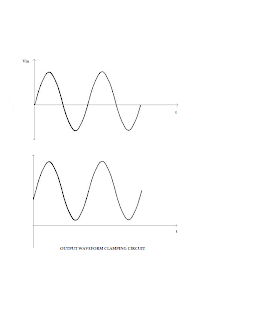# OP AMP CLAMPING CIRCUIT

In clamper circuits a predetermined dc level is added to the output voltage. In other words, the output is clamped to a desired dc level. If the clamped dc level is Positive, the clamper is a POSITIVE CLAMPER. On the other hand, if the clamped dc level is negative, the clamper is called a NEGATIVE CLAMPER. Other equivalent terms used for clamper are dc inserter or restorer. Recall that op amps are designed to operate from a single power supply, such as the 124 series, employ a dc insertion technique. The inverting operational amplifier (op amp) that use this technique is shown in the circuit diagram.
The value of the capacitors in these circuits depends on the different input rates and pulse widths. It is also noticed that the output waveforms have an added dc level, which is approximately equal to Vcc/2. This fixed positive dc level is needed to obtain a maximum undistorted symmetrical sine wave. The output voltage of the peak clamper is a net result of ac and dc input voltages applied to the -ve and +ve input terminals, respectively. Therefore, to understand the circuit operation, each input must be considered separately.
First consider the input voltage Vref at the positive input. Since this voltage is positive Vo is also positive, which forward biases the diode D1. This closes the feedback loop and the opamp operates as a voltage follower. This is possible because c1 is an open circuit for dc voltage. Therefore, Vo=+Vref. As far as voltage Vin at the negative input is concerned, during its negative half cycle, diode D1 conducts, charging C1 to the negative peak value of Vp. However, during the positive half cycle of Vin diode D1 is reverse biased; hence, the voltage Vp across the capacitor acquired during the negative half cycle is retained. Since this voltage Vp is in series with the positive peak voltage Vp, the output voltage Vo=2Vp.### Tittu

#### Author & Editor

I'm Tittu Thomas, An Electronics and Communication Engineer from India, Kerala. I love doing hobby electronics circuits, blogging, programming, etc. Started this blog while doing my B-Tech degree under CUSAT university.